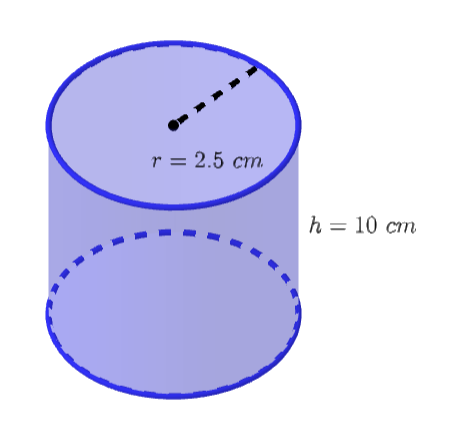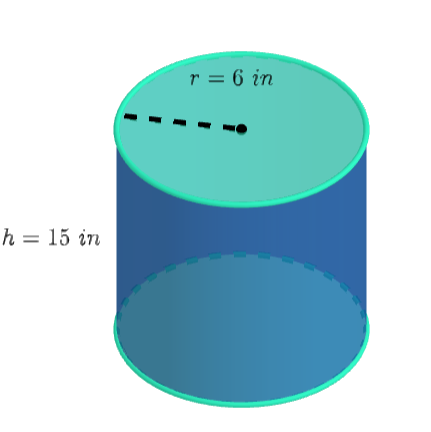Surface Area of a Cylinder homework Help at TutorEye

# Best Homework Help For Surface Area of a Cylinder

## Top Questions

t schedule 3 people for the day my % scheduled is 78.57% My question is how did the computer come up with the 78.57%?
View More

I would love to get a written solution. I did the other proof tree that you can see in the attaached files. So its supposed to look like that Thank You.
View More

xtbook. i really need in in half an hour
View More

## Surface Area of a Cylinder:

The surface area of a cylinder refers to the sum of areas covered by its parallel base and its lateral surface area. Lateral surface area is the area covered by its curved surface. This area is expressed as square units. Some standard units of surface area are square inches, square feet, square meters, square centimeters, etc.

 Lateral Surface Area 2πrh Total Surface Area 2πrh + 2πr2 = 2πr (h+r)

## Surface Area of a Cylinder Sample Questions:

Question 1: Calculate the lateral surface area of the cylinder having a radius of 10 cm and a height of 15 cm. Use π=3.14

Explanation: Plug the values in the lateral surface area of cyclinder formula.

Question 2: Calculate the lateral surface area of the following cylindrical can:Explanation: Plug the value of radius and height in the surface area of cyclinder formula.

Question 3: Calculate the total area of tin sheet needed to manufacture the following paint bucket.Explanation: Plug the value of radius and height in the total surface area of cyclinder formula.

Question 4: Calculate the total surface area of the cylinder having a diameter of 12 cm and a height of 9 cm. Use π=3.14

Explanation: Plug the value of radius and height in the total surface area of cyclinder formula.

Question 5: Find the total surface area of the cylindrical disc having a diameter of 22 in and a thickness of 3 in.

Explanation: Plug the value of radius and height in the total surface area of cyclinder formula.

Question 6: The surface area of a cylindrical tank is 747.7 ft2. Find the height of the cylinder, given the base radius is 7 ft.

Explanation: Plug the value of radius and total surface area in the total surface area of cyclinder formula.

Question 7: The lateral surface area of a cylindrical tank is 345.4 ft2. Find the top radius of the cylinder, given the height is 11 ft.

Explanation: Plug the values and then isolate the the variable.

Question 8: Calculate the total cost of the tin sheet, which is required to manufacture 10 identical cylinders having a radius of 15 in with a height of 20 in. Given the price of the sheet is 6 USD per square foot.

Explanation: Plug the values in the total surface area of cyclinder formula. Multiply the answer with 10 and then find the area in the square root.

Question 9: Find the radius of the cylinder having a total surface area of 1413.72 cm2, with 16 cm height.

Explanation: Plug the value of height and total surface area in the total surface area of cyclinder formula.

Question 10: Find the radius of the cylinder having a total surface area of 904.78 in2, with 10 in height.